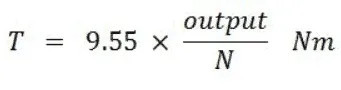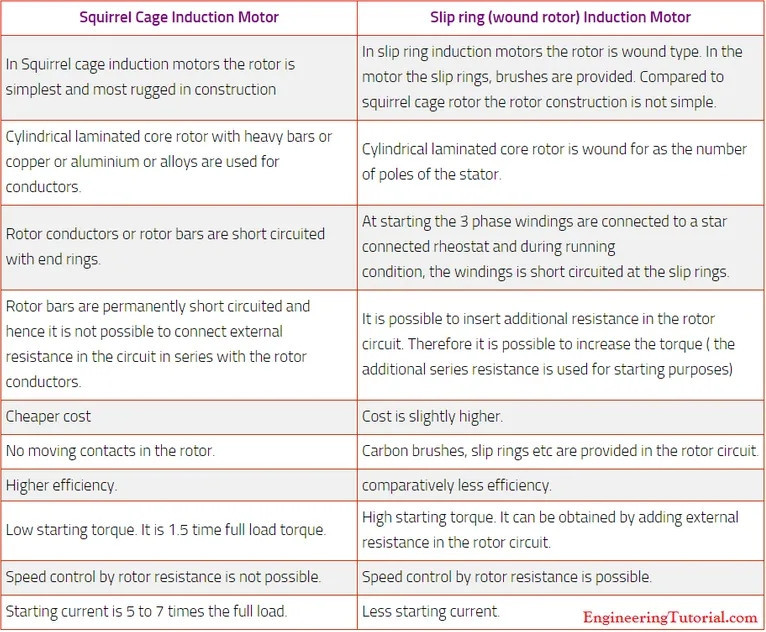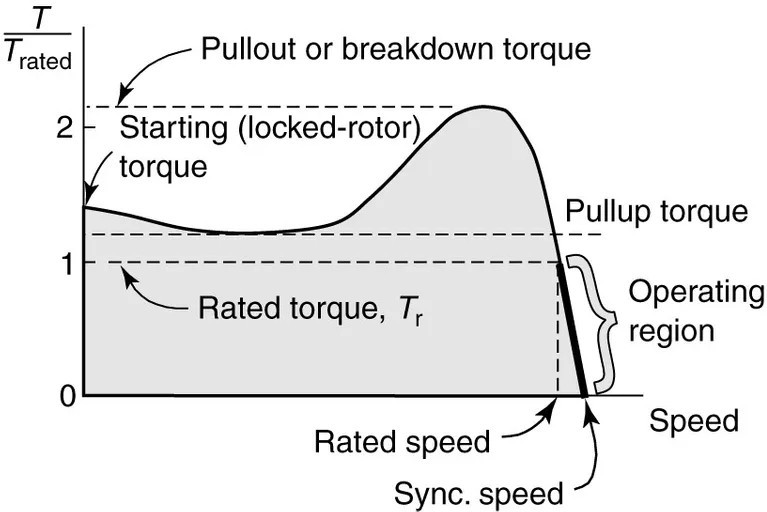# Induction Motors Questions

Here are the most frequently asked questions on Induction motors. This article aims to cover most of the interview questions on induction motors so as to prepare you to crack a job interview.

## Explain the principle of operation of induction motors.

The induction motors have three-phase winding at the stator similar to that in synchronous machines. When a three-phase voltage is applied to the stator coils, a rotating magnetic field is formed. This varying magnetic field comes in contact with the rotor conductors and induces EMF in it.

The ends of rotor winding are short-circuited so that there flows a current proportional to the induced EMF. Due to the current flow, another magnetic field rotating in the direction same as that of the stator is set up. The interaction between these two magnetic fields generates a torque that tends to rotate the motor in the direction of the rotating magnetic field of the stator. Induction motors are self-starting motors.

## What are the types of induction motors?

Based on the construction, induction motors are classified into: Squirrel cage induction motor and Slip ring induction motor. Depending on the input power supply, they can be classified into single-phase and three-phase induction motors.

## What are the parts of an induction motor?

• Stator
• Rotor
• Commutator
• Bearing and shaft assembly
• Insulation materials
• Casing, brushes, and terminals

## How can you control the speed of an induction motor?

The following are the different techniques adapted to control the speed of an induction motor:

1. Pole changing method.
2. Stator voltage control method.
3. Frequency control method.
4. Rotor resistance control method (Slip ring motors).

Nowadays, Variable Speed drives are used to control the speed of induction motors. It controls the input voltage and supply frequency to accurately control motor speed.

## The formula for motor torque## List the differences between a squirrel cage induction motor and a slip ring induction motor.## What are the techniques used to start an induction motor?

1. Direct-on-line starter.
2. Star-Delta Starter.
3. Autotransformer Starter.
4. Soft Starter.
5. Using Variable frequency drives.

## Draw torque-speed characteristics of Induction motor## What is breakdown torque?

It is the maximum torque the motor can produce.

## What is pull-up torque?

Pull Up Torque is the minimum torque developed by the motor as it accelerates from standstill to the speed at which breakdown torque occurs.

## What is rated torque?

The rated torque of an induction motor is the maximum torque it can deliver to the load at its rated speed.

## Why is an induction motor called an asynchronous motor?

The speed of the rotation of the rotor is always less than the synchronous speed of the applied stator voltage. Moreover, the rotor can never match the speed of the rotating magnetic field. Hence these motors are known as asynchronous motors.

## What are the basic protections required for an induction motors?

2. Short Circuit protection.
3. Protection against Phase failures.
4. Overvoltage protection.
5. Earth fault protection.

## What is meant by slip in induction motors?

The per-unit difference between the synchronous speed and the actual rotor speed is known as slip.

## List out the motor efficiency standards

• IE1 – Standard Efficiency Class
• IE2 – High Efficiency